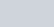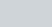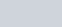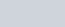If three consecutive vertices of a parallelogram are
Question:

If three consecutive vertices of a parallelogram are (1, −2), (3, 6) and (5, 10), find its fourth vertex.

Solution:

Let ABCD be a parallelogram in which the co-ordinates of the vertices are A (1,−2);

B (3, 6) and C(5, 10). We have to find the co-ordinates of the forth vertex.

Let the forth vertex beSince ABCD is a parallelogram, the diagonals bisect each other. Therefore the mid-point of the diagonals of the parallelogram will coincide.

Now to find the mid-pointof two pointsandwe use section formula as,

$\mathrm{P}(x, y)=\left(\frac{x_{1}+x_{2}}{2}, \frac{y_{1}+y_{2}}{2}\right)$

The mid-point of the diagonals of the parallelogram will coincide.

So,

Co-ordinate of mid-point of $A C=$ Co-ordinate of mid-point of $B D$

Therefore,

$\left(\frac{5+1}{2}, \frac{10-2}{2}\right)=\left(\frac{x+3}{2}, \frac{y+6}{2}\right)$

$\left(\frac{x+3}{2}, \frac{y+6}{2}\right)=(3,4)$

Now equate the individual terms to get the unknown value. So,

$\frac{x+3}{2}=3$

$x=3$

Similarly,

$\frac{y+6}{2}=4$

$y=2$

So the forth vertex is $\mathrm{D}(3,2)$